####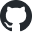https://github.com/rsharp-lang/R-sharp## ROC介绍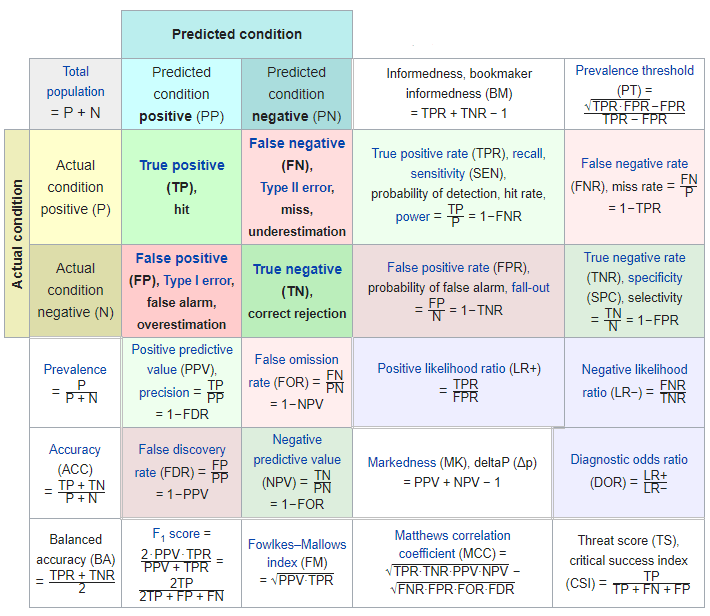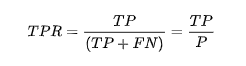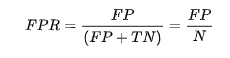Dim TP As Integer
Dim FP As Integer
Dim TN As Integer
Dim FN As Integer
Dim All%

For Each n As T In entity
Dim validate = getValidate(n)
Dim predict = getPredict(n)

If validate = True Then
If predict = True Then
TP += 1
Else
FN += 1
End If
Else
If predict = True Then
FP += 1
Else
TN += 1
End If
End If

All += 1
Next

### AUC值计算

AUC（Area Under Curve）的本质含义反映的是对于任意一对正负例样本，模型将正样本预测为正例的可能性大于将负例预测为正例的可能性的概率。我们可以从AUC的英文全称知道，其含义为曲线下方的面积，很明显，当AUC的面积越大，我们的分类器的假阳性越低，真阳性率越高。AUC值的一个简单的计算方法就是将计算ROC得到的不同阈值下的由TPR和FPR这两个指标构成的坐标所产生的无数个小矩形的和。

0.9 ~ 1.0（优秀）
0.8 ~ 0.9（良好）
0.7 ~ 0.8（一般）
0.6 ~ 0.7（很差）
0.5 ~ 0.6（无法区分）

## 在R语言中进行ROC分析

library(ROCR);

# predictions为预测标签，labels为真实标签
pred <- prediction(predictions, labels);
perf <- performance(pred, "tpr", "fpr");
auc  <- performance(pred, 'auc');
auc  <- unlist(slot(auc,"y.values"));

plot(perf,
xlim = c(0,1),
ylim = c(0,1),
col  = 'red',
main = paste("ROC curve (", "AUC = ",auc,")"),
lwd  = 2,
cex.main = 1.3,
cex.lab  = 1.2,
cex.axis = 1.2,
font     = 1.2
);
abline(0, 1);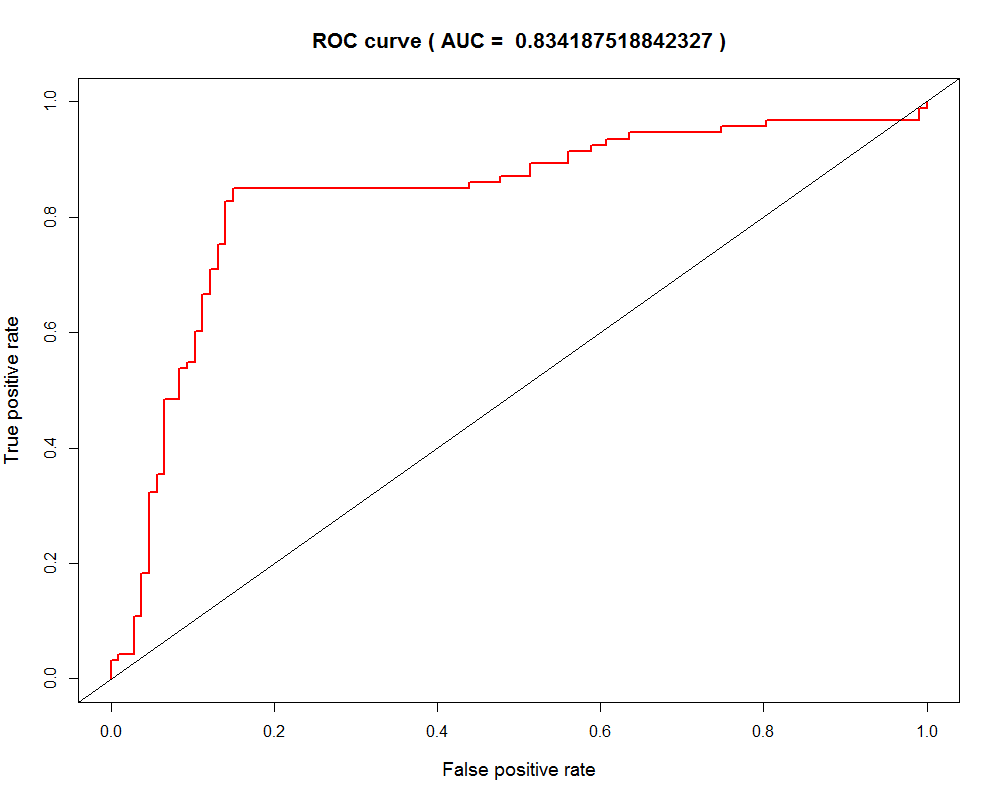ROC曲线越凸越近左上角表明其诊断价值越大，利于不同指标间的比较。曲线下面积可评价诊断准确性。

## 在R#语言中进行ROC计算分析

• prediction 这个函数的作用与R语言之中的ROCR程序包中的prediction功能是一样的。就是接受一个预测的结果向量和一个真实的标签结果向量计算出混淆矩阵结果。
• AUC 这个函数的作用，通过其名字就可以看得出来，是计算ROC分析中的AUC值的。其接受的计算参数来自于prediction函数的参数返回值。
imports "validation" from "MLkit";

# predictions is the score value of the predictions
# and labels is the real data labels, should be a logical vector that
# equals to predictions vector in size
const pred <- prediction(predictions, labels);
const auc  <- AUC(pred);

const url = "github://rsharp-lang/R-sharp/blob/master/Library/demo/machineLearning/umap/ROC.csv";
const data = read.csv(file = url);

str(data);

# 'data.frame':   200 obs. of  2 variables:
#  $V1 : num [1:200] 0.6125478 0.364271 0.4321361 0.1402911 0.3848959 0.2444155 ... #$ V2 : int [1:200] 1 1 0 0 0 1 ...
#
#         V1          V2
# <mode>  <double>    <integer>
# [1, ]    0.6125478  1
# [2, ]    0.364271   1
# [3, ]    0.4321361  0
# [4, ]    0.1402911  0
# [5, ]    0.3848959  0
# [6, ]    0.2444155  1

const pred = prediction(data[, 1], data[, 2]);

print(AUC = ${AUC(pred)}); #  "AUC = 83.4187518842326" bitmap(file = ${dirname(@script)}/ROC.png) {
plot(pred);
}const simple_auc <- function(TPR, FPR){
# inputs already sorted, best scores first
const dFPR <- c(diff(FPR), 0);
const dTPR <- c(diff(TPR), 0);

sum(TPR * dFPR) + sum(dTPR * dFPR) / 2;
};

print(simple_auc(
TPR = pred$sensibility, FPR = pred$FPR
));
#   83.4187519

Public Function SimpleAUC(TPR As Vector, FPR As Vector) As Double
Dim dFPR As Vector = C(diff(FPR), 0)
Dim dTPR As Vector = C(diff(TPR), 0)

Return (TPR * dFPR).Sum + (dTPR * dFPR).Sum / 2
End Function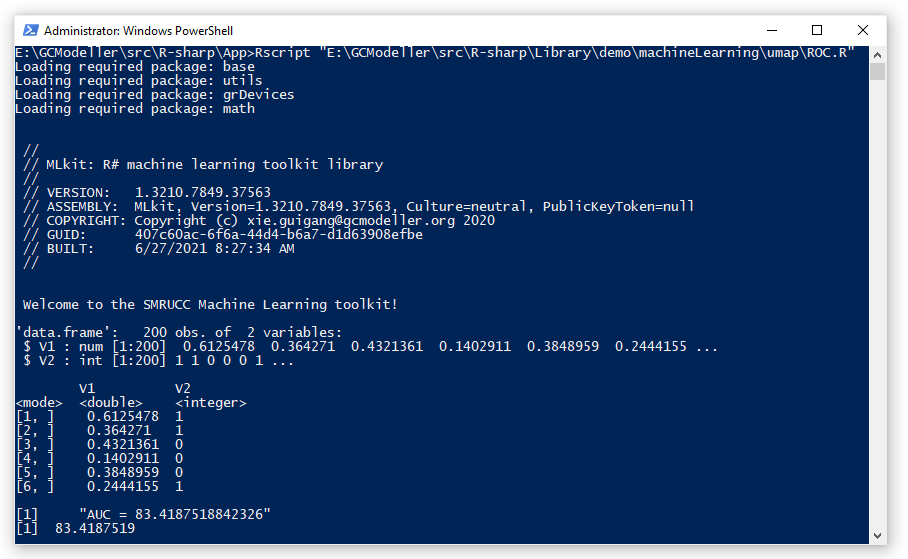Latest posts by 谢桂纲 (see all)

### Order by Date Name Attachments

#### One response

1. […] 从之前的测试结果脚本中，我们将预测结果与对应的真实标签都放在一起了。现在我们可以基于这两个向量数据进行XGBoost分类器的分类性能ROC曲线的可视化： […]

来自中国
1.2.3.4.空间Spot结果在下载到的mzkit文件夹中有示例吗？我试了试，不是10X结果中的tissue_positions_list.csv（软件选择此文件，直接error）；在默认结果中也没找到类似的文件；

5.